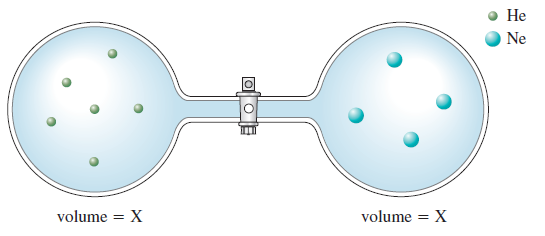# Problem: Consider the flasks in the following diagrams.b. Assuming the connecting tube has negligible volume, draw what each diagram will look like after the stopcock between the two flasks is opened.

###### FREE Expert Solution

after the stopcock between the two flasks is opened → molecules will move to balance the pressures (equilibrium)

assuming one sphere = 1 mole of gas

88% (305 ratings)###### Problem Details

Consider the flasks in the following diagrams.b. Assuming the connecting tube has negligible volume, draw what each diagram will look like after the stopcock between the two flasks is opened.

Frequently Asked Questions

What scientific concept do you need to know in order to solve this problem?

Our tutors have indicated that to solve this problem you will need to apply the Partial Pressure concept. You can view video lessons to learn Partial Pressure. Or if you need more Partial Pressure practice, you can also practice Partial Pressure practice problems.

What professor is this problem relevant for?

Based on our data, we think this problem is relevant for Professor Leveritt's class at UIUC.

What textbook is this problem found in?

Our data indicates that this problem or a close variation was asked in Chemistry: An Atoms First Approach - Zumdahl Atoms 1st 2nd Edition. You can also practice Chemistry: An Atoms First Approach - Zumdahl Atoms 1st 2nd Edition practice problems.